Select a problem set using the buttons above, then use your mouse or tab key to select a question. Fill in the blank with the correct answer for each problem. When you have answered all of the questions, ask Charlie how you did.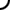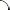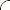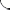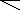Identify the property being illustrated by each statement as either associative or commutative. Watch your spelling.   5 + 4 = 4 + 5 2 + (3 + 7) = (2 + 3) + 7 5 + (1 + 2) + 4 = 5 + 1 + (2 + 4) 8 + (9 + 4) = 8 + (4 + 9) 15 + 7 = 7 + 15 (2 + 3) + 6 = 2 + (3 + 6)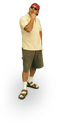Identify the property being illustrated by each statement as either associative or commutative. Watch your spelling.   (8 + 7) + 3 = 8 + (7 + 3) 4 + (9 + 1) = 4 + (1 + 9) (2 + 4) + 3 + 11 = 2 + 4 + (3 + 11) (5 + 2) + 7 = 5 + (2 + 7) 6 + 10 = 10 + 6 (5 + 8) + 9 = 9 + (5 + 8)Identify the property being illustrated by each statement as either associative or commutative. Watch your spelling.   5 + (10 + 3) = 5 + (3 + 10) 8 + 1 = 1 + 8 2 + (3 + 4) = (2 + 3) + 4 3 + 0 + (7 + 5) = 3 + 0 + (5 + 7) 6 + (7 + 9) = (6 + 7) + 9 4 + (3 + 2) + 8 = (4 + 3) + 2 + 8